#### How to Use IF Function in Excel

You can learn here, what IF function is and how to use it.

## IF Function Basics

IF function is one which are used the most often in Excel. IF is a logical function. But you can not only check TRUE / FALSE values.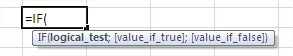Let’s take a look at some IF function examples. IF Function contains from 3 parts:

1. Logical test – IF function checks if this test is true or false in the cell
2. Value if true – IF function puts this value if logical test is true
3. Value if false – IF function puts this value if logical test is false

## How does the IF function work?

The IF function works by first evaluating the condition. If the condition is true, the IF function returns the first value. If the condition is false, the IF function returns the second value.

## If function examples

Here you have some examples:

### IF exam is passed?

#### Basic example

The simpliest example is to make a table with exam results. In this table Excel will show, if the Student passed the exam.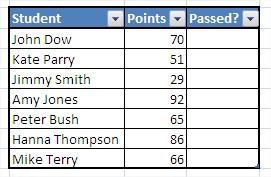Thanks to IF function you can automatically check, if the student passed the exam. The formula is:

=IF(\$C3>50,”PASSED”,”FAILED”)

IF formula checks the logical test, which is if the student got more points than 50. If logical test is true, Excel writes PASSED. If student got less then 50 points Excel writes FAILED.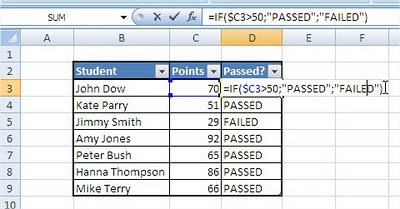Isn’t it simple?

#### More difficult example

Let’s do something more difficult. You want to check if the student passed the exam and you want to get the mark.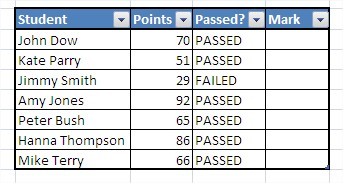A is more than 90 points. B is more than 75 points. C is more than 60 points. D is more than 50 points.

It is not hard to do. You must to do more logical tests in Excel’s formula.

You check if it is more than 90 points. If it is more, mark is A. If it is not, check if more than 75 points… If all logical tests aren’t true, the mark is E. Formula is:

=IF(\$C3>90,”A”,IF(\$C3>75,”B”,IF(\$C3>60,”C”,IF(\$C3>50,”D”,”E”))))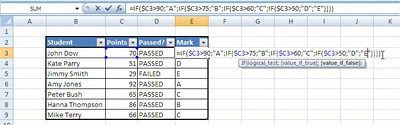If formula seems to be complicated, but it is easy to get it. And the effect is magnificent.

## Key Takeaways

• The IF function is a logical function that can be used to check if a condition is met and return a different value depending on the outcome.
• The IF function has three arguments: the logical test, the value if true, and the value if false.
• The logical test can be any expression that evaluates to TRUE or FALSE.
• The value if true and the value if false can be any value, including text, numbers, or formulas.

## FAQ

• Q: What is the difference between the IF function and the AND function?
• A: The IF function checks if a single condition is met, while the AND function checks if multiple conditions are met.
• Q: Can I use the IF function with other functions?
• A: Yes, you can use the IF function with other functions, such as the SUM function, the COUNT function, and the MAX function.